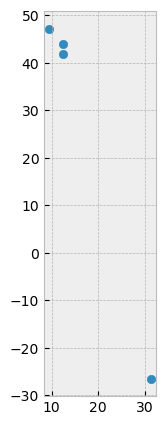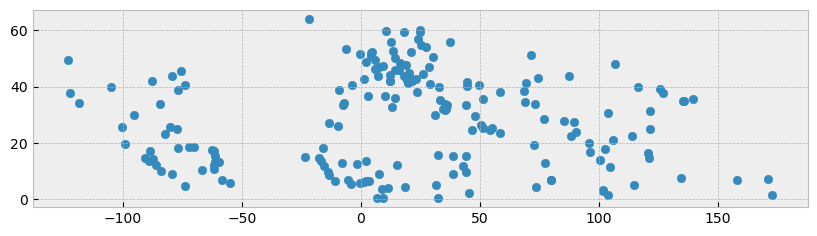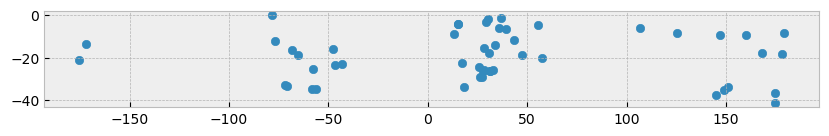Learning Objectives

• Create and manipulate vector attributes

• Subset data

• Plot lat lon as points

• Subset points by location

Review

# Attributes & Indexing for Vector Data#Fig. 52 Structure of a GeoDataFrame extends the functionality of a Pandas DataFrame#

Each GeoSeries can contain any geometry type (e.g. points, lines, polygon) and has a GeoSeries.crs attribute, which stores information on the projection (CRS stands for Coordinate Reference System). Therefore, each GeoSeries in a GeoDataFrame can be in a different projection, allowing you to have, for example, multiple versions of the same geometry, just in a different CRS.

Tip

## Create New Attributes#

One of the most basic operations is creating new attributes. Let’s say for instance we want to look at the world population in millions. We can start with an existing column of data pop_est. Let’s start by looking at the column names:

import geopandas
world.columns

Index(['pop_est', 'continent', 'name', 'iso_a3', 'gdp_md_est', 'geometry'], dtype='object')


We can then do basic operations on the basis of column names. Here we create a new column m_pop_est:

world['m_pop_est'] = world['pop_est'] / 1e6

pop_est continent name iso_a3 gdp_md_est geometry m_pop_est
0 889953.0 Oceania Fiji FJI 5496 MULTIPOLYGON (((180.00000 -16.06713, 180.00000... 0.889953
1 58005463.0 Africa Tanzania TZA 63177 POLYGON ((33.90371 -0.95000, 34.07262 -1.05982... 58.005463

## Indexing and Selecting Data#

GeoPandas inherits the standard pandas methods for indexing/selecting data. This includes label based indexing with .loc and integer position based indexing with .iloc, which apply to both GeoSeries and GeoDataFrame objects. For more information on indexing/selecting, see the pandas documentation.

### Selection by Index Position#

Pandas provides a suite of methods in order to get purely integer based indexing. The semantics follow closely Python and NumPy slicing. These are 0-based indexing. When slicing, the start bound is included, while the upper bound is excluded. For instance name = 'fudge' with name[0:3] returns 'fud', where f is at 0 and g is at the 3 position with the upper bound excluded.

import matplotlib.pyplot as plt
plt.style.use('bmh') # better for plotting geometries vs general plots.

northern_world = world.iloc[ 0:4 ]
northern_world.plot(figsize=(10,5))
plt.show()#### Different choices for indexing#

Object selection has had a number of user-requested additions in order to support more explicit location based indexing.

Getting values from an object with multi-axes selection uses the following notation (using .loc as an example, but the following applies to .iloc as well). Any of the axes accessors may be the null slice :. Axes left out of the specification are assumed to be :, e.g. p.loc['a'] is equivalent to p.loc['a', :, :].

Object Type

Indexers

Series

s.loc[indexer]

DataFrame

df.loc[row_indexer,column_indexer]

### Subset Points by Location#

In addition to the standard pandas methods, GeoPandas also provides coordinate based indexing with the cx indexer, which slices using a bounding box. Geometries in the GeoSeries or GeoDataFrame that intersect the bounding box will be returned.

Using the world dataset, we can use this functionality to quickly select all cities in the northern and southern hemisphere using a _CoordinateIndexer using .cx. .cx allows you to quickly access the table’s geometry, where indexing reflects [x,y] or [lon,lat]. Here we will query points above and below 0 degrees latitude:

world = geopandas.read_file(geopandas.datasets.get_path('naturalearth_cities'))
northern_world = world.cx[ : , 0: ]   # subsets all rows above 0 with a slice
northern_world.plot(figsize=(10, 5))
plt.show()world = geopandas.read_file(geopandas.datasets.get_path('naturalearth_cities'))
southern_world = world.cx[ : , :0 ]   # subsets all rows below 0  with a slice
southern_world.plot(figsize=(10, 5))
plt.show()